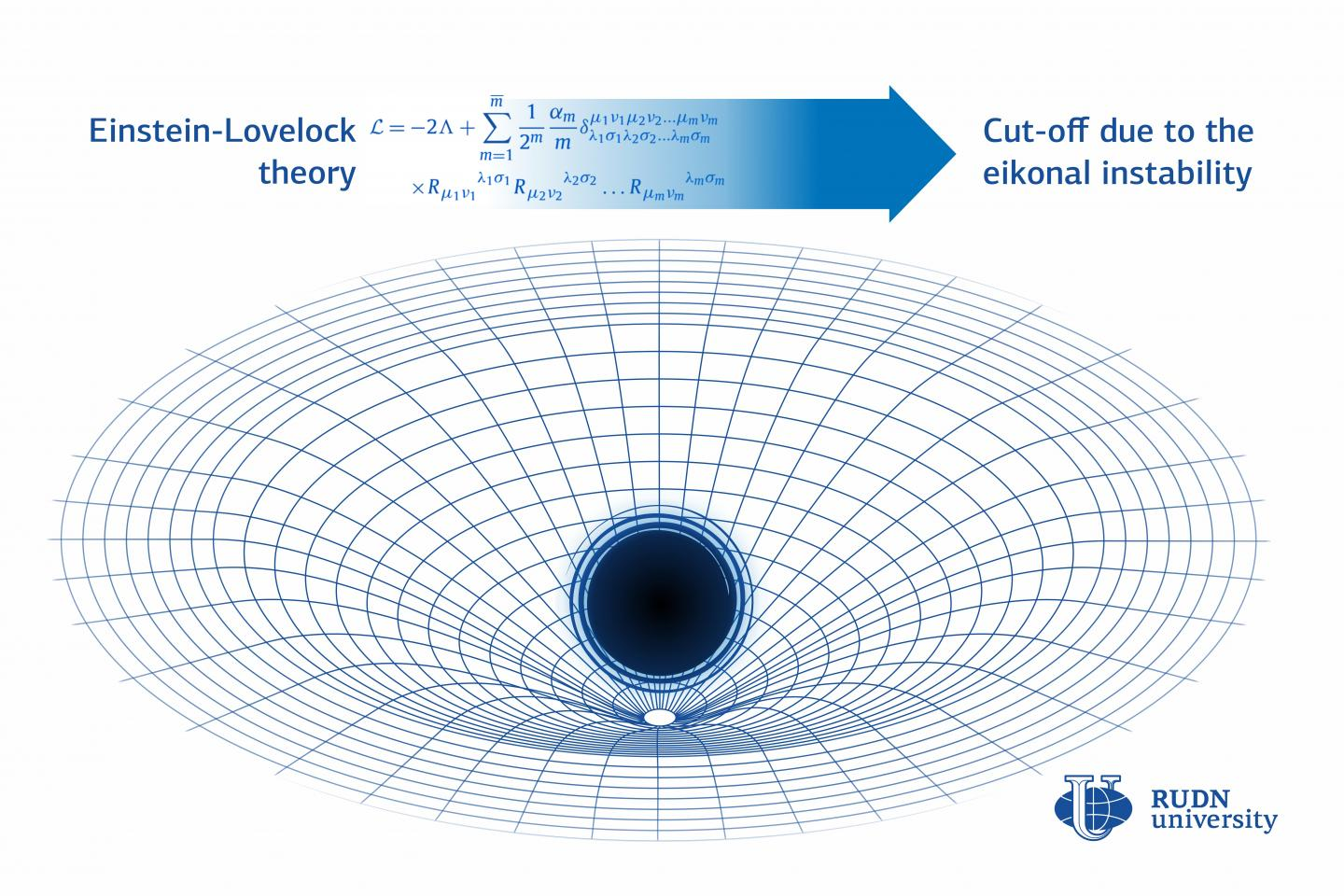News Release

# A RUDN University physicist simplified the Einstein-lovelock theory for black holes

Peer-Reviewed Publication

RUDN Universityimage: Allowing for quantum corrections, the Einstein-Lovelock theory describes black holes with an equation that contains an infinite number of terms. However, according to a RUDN University physicist, the geometry of a black hole in this theory can be presented in a compact form, and a limited number of terms can suffice to describe the observed values. This could help scientists study black holes in theories with quantum corrections to Einstein's equations. view more  Credit: RUDN University

Allowing for quantum corrections, the Einstein-Lovelock theory describes black holes with an equation that contains an infinite number of terms. However, according to a RUDN University physicist, the geometry of a black hole in this theory can be presented in a compact form, and a limited number of terms can suffice to describe the observed values. This could help scientists study black holes in theories with quantum corrections to Einstein's equations. The work was published in the Physics Letters B journal.

Einstein's general theory of relativity predicted the existence of black holes--supermassive objects in the Universe that attract everything, including light. Black holes are described by many mathematical models, one of which is the Einstein-Lovelock theory that imposes quantum corrections to elaborate on the general theory of relativity. In it, a black hole is described by a sum of an infinite number of terms. However, a physicist from RUDN University confirmed that a limited number of terms can suffice to describe the effects observed in the vicinity of a black hole. Other components of the equation have a negligibly small contribution that can be ignored. This would considerably simplify calculations and help researchers study black holes in theories with quantum corrections.

According to Einstein's theory, heavy objects warp space-time--a 4D construction that has three spatial and one temporal dimension. In 1971, Lovelock generalized this theory to include any number of dimensions. The Einstein-Lovelock equation is an infinite sum: the first two terms in it are Einstein's representation, and each subsequent one details the space-time curvature.

Each term in the Einstein-Lovelock equation is multiplied by the so-called coupling constant. According to the physicist from RUDN University, if one sticks to the positive values of coupling constants, high curvature corrections can be 'cut off'. This is due to the fact that each coupling constant has a critical value: after it is reached, a black hole becomes unstable, i.e. unable to exist in reality. Such a representation is still possible from the point of view of mathematics but has no physical sense. The more terms, the lower is the critical value for coupling constants. Therefore the stability of a black hole (i.e. the possibility of its physical existence) can be used as a criterion to remove redundant terms.

"With every new Lovelock's term, the critical value of coupling constants becomes lower. This is an important observation: it confirms that in order to find the biggest possible correction to black hole geometry caused by a newly added Lovelock's term, all other terms can be considered negligibly small," said Roman Konoplya, a researcher at the Academic Research Institute for Gravitation and Cosmology, RUDN University.

According to the scientist and his team, the main observable values (such as the radius of a black hole shadow) remain virtually unchanged when the Lovelock corrections of higher than the fourth order in curvature are included. These findings can be useful not only for studying processes in the black holes but also for confirming theoretical predictions associated with possible generalizations of Einstein's theory.

###

Disclaimer: AAAS and EurekAlert! are not responsible for the accuracy of news releases posted to EurekAlert! by contributing institutions or for the use of any information through the EurekAlert system.